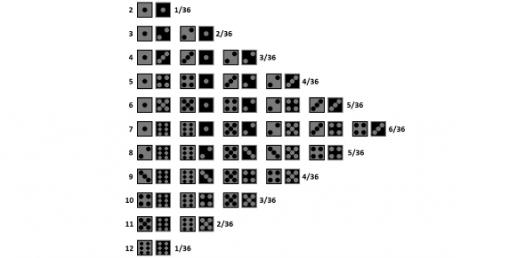# Quiz: Take The Probability Practice Questions!

5 Questions | Total Attempts: 713SettingsWhat do you know about probability? You can answer SAT math probability word problems by following these simple steps: Identify events for which probability is to be verified. Calculate the total number of possible outcomes, estimate the probability of each event. Add the probability of each event. Once you have practiced probability long enough, take the quiz to test your knowledge.

• 1.
You choose a letter from the alphabet at random.  What is the probability of choosing the letter Q?
• A.

1 out of 2

• B.

1 out of 26

• C.

1 out of 12

• D.

0 out of 1

• 2.
There are 12 marbles in a bag.  Four are yellow, two are green, and 6 are red.  What is the probability of choosing a yellow marble?
• A.

33.333%

• B.

1/3

• C.

4 out of 12

• D.

All of the above.

• 3.
You are looking at a menu with 4 main courses, 2 side dishes, 3 vegetables, 2 desserts, and 2 beverages.  How many combinations can you make if you choose one of each?
• A.

96

• B.

48

• C.

5

• D.

13

• 4.
Suppose you flip a coin 3 times, what is the probability that the coin will land on heads 3 times?
• A.

1/2

• B.

1/6

• C.

1/4

• D.

1/8

• 5.
Using your SRB, look at the Venn diagram in the middle of page 263 (Ms. Waller's students).  If Ms. Waller had a new student added to her Music class, how would the Venn Diagram change?
• A.

The 17 would become 18.

• B.

The 4 would become 5.

• C.

The 10 would become 11.

• D.

Nothing would change at all.

Related TopicsBack to top